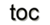Next: Bibliography Up: 4 Applied Concepts Previous: Appendix B: STL Iterator Analysis

# Appendix C: Cell Properties of Discretization Schemes

As introduced in Section 2, the finite volume and finite element methods use different cell types for their approximations. Finite volumes write the balance equations in terms of global quantities, whereas finite elements, making use of spread cells with spread boundaries, is forced to use field functions defined locally over the whole domain. Despite this difference, neither finite volume nor finite element discretize the operator representing the local version of the balance equation. Instead they both resort to a global version since a topological equation directly applies to regions with finite extension. A detailed geometrical analysis  based on the identification of the weighting functions was given.

The weighted residual formulation of the finite element method starts from the continuous formulation of a weighted domain: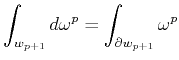(13.1)

The relationship between the two concepts, the continuous concept of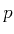-differential forms (Section 1.7) and the corresponding weighted domains, and the discrete concept related to chains and cochains (Section 1.4) can be used to algebraically handle the cells from a cell complex and the discrete projection of a physical field. The weighted domain and the-form are then directly related to the-chains and-cochains. When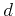is used as a divergence operator, the following expression is obtained in the discrete case: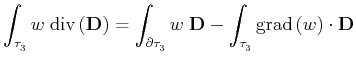(13.2)

where the 3-cell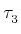can be taken as the support of the weight function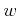. With the expression for the boundary of a weighted three-dimensional geometric object, the following formal definition can be obtained: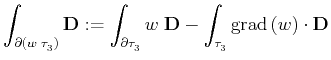(13.3)

where the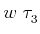represents a weighted 3-cell. This "boundary" includes actually an integral of the whole 3-cell, and not only on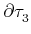, except in the particular case of a constant weight function on its supporting cell. A corresponding interpretation of the weight functionas a continuous counterpart of a chain yields a concept called spread cell, compared to a "crisp" cell, considered so far by a constant weight function. If a weight function is constant on a cell and zero outside, the second term of the equation vanishes and the finite element method corresponds with a finite volume method. Due to the fact that these spread cells overlap, this assembly of cells does not model the concepts introduced for a consistent cell complex.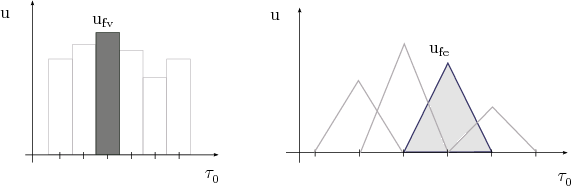Figure C.1 depicts the different approaches of the finite volume and finite element method. The x-axis is divided into one-dimensional cells, marked with vertical lines. The finite volume method uses crisp cells which are aligned accordingly with the dual complexes of the given cells of the underlying cell complex. The finite element method uses spread cells which do not build a consistent cell complex. In this example, each cell, depicted by the basis function, is spread over two cells. From the point of view of the dual complex, which is not used by the finite element method, the cells extend beyond the area of a dual cell. The important part of the integration by parts in the FE method can be thereby explained with the necessity to operate with the boundary of the FEM spread cells in order to express topological equations.Next: Bibliography Up: 4 Applied Concepts Previous: 12. STL Iterator Analysis

R. Heinzl: Concepts for Scientific Computing